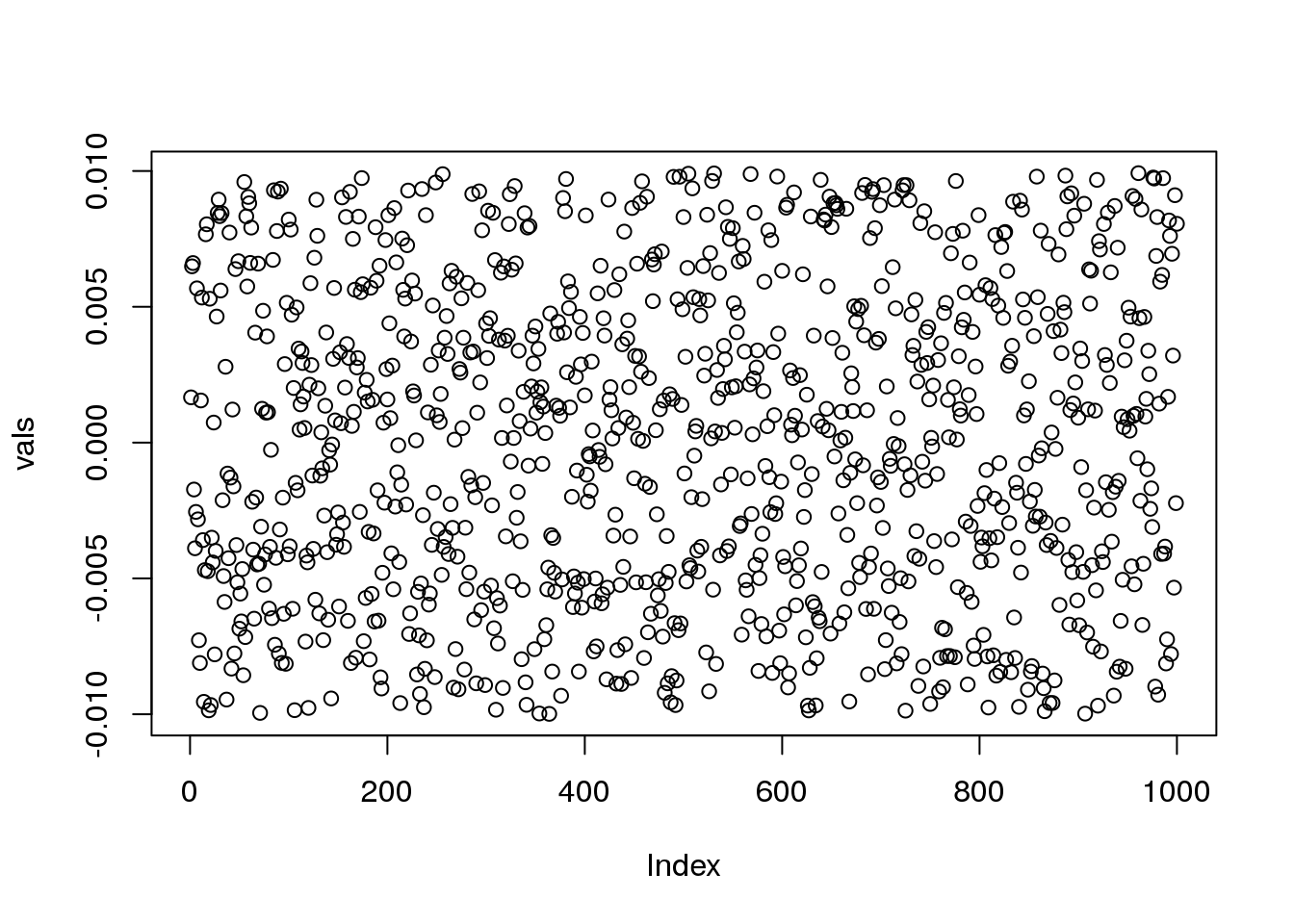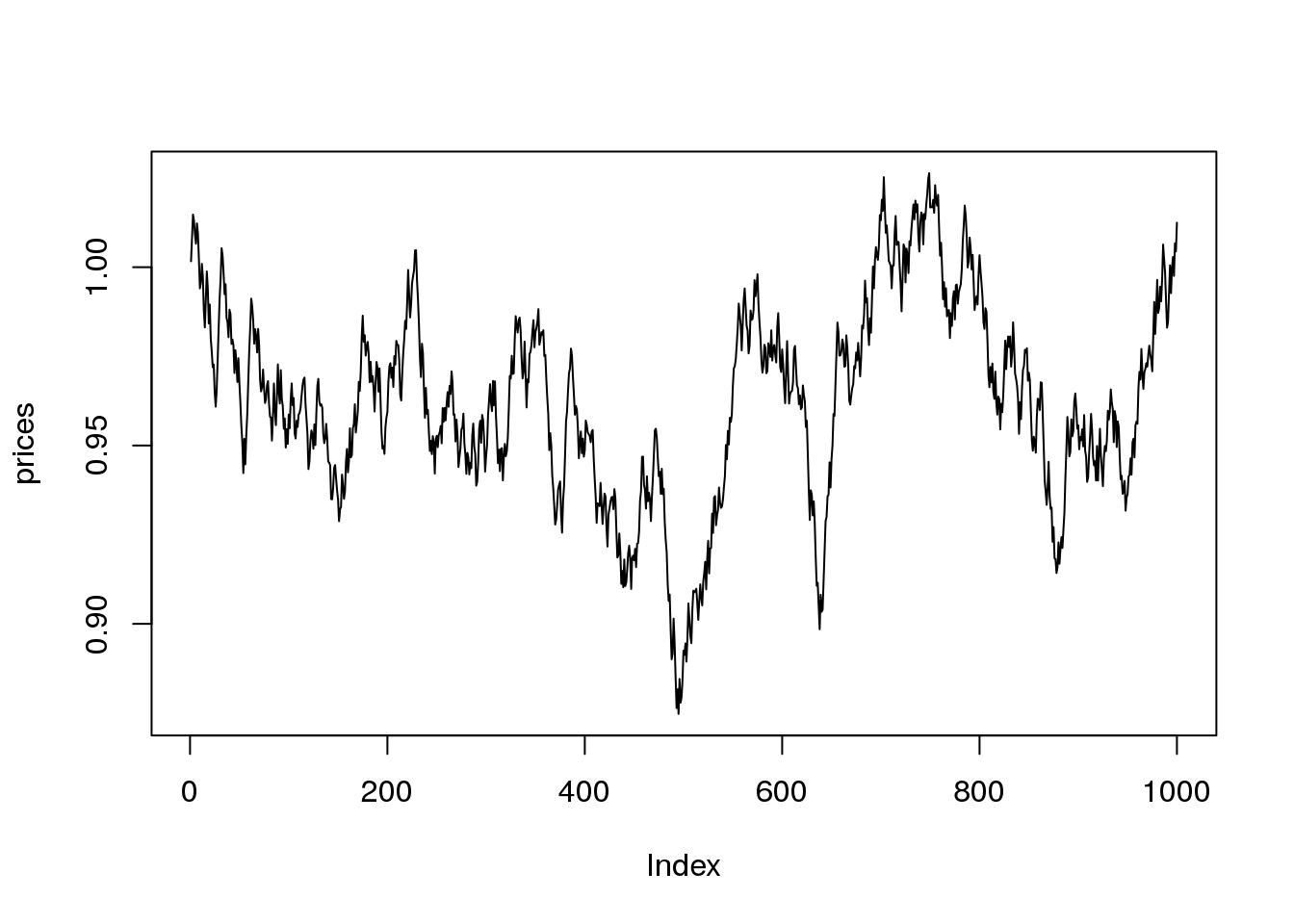# Introduction

In this study, we want to investigate the possibilities to use the random walk behaviors in finance to generate a profitable strategy.

## Simple random returns

We want to consider the currency prices on the FOREX market as random variables described by a random walk. So if we consider a given currency pair, for instance EURUSD, we have price series defined as $${p_1, p_2, \dots, p_t}$$. For now we just assume we will use a constant time delta between subsequent prices. And the change between subsequent prices $$\Delta p_t = p_t - p_{t-1}$$ is random.

Now the question would be: what kind of randomness do we really have here ?

Let’s start with the most simple option, and consider the price changes are simple uniform random values:

vals <- runif(n = 1000, min = -0.01, max = 0.01)
head(vals)
##   0.001665592  0.006489158  0.006610746 -0.001727523 -0.003891034
##  -0.002551775
plot(vals)The first thing we can notice here which is wrong about this simple model is that here we place an hard limit on the possible change from one price to another: given the previous random uniform sampling we have $$|\Delta p_t| \le 0.01$$ which is totally arbitrary and certainly doesn’t make much sense when trying to model a price series. But anyway, we can build the corresponding price series starting from an arbitrary price value to see what it would look like:

initPrice = 1.0
changes <- cumsum(vals)
prices <- initPrice + changes

plot(prices, type = "l")So, this really simple model already given us somewhat good looking price series as we can see above. But let’s refine this a little bit further and now rather assume the price changes are normally distributed, mean $$\mu = 0.0$$ and standard deviation $$\sigma = 0.0033$$ so that we roughtly keep most of the samples in the range [-0.01, 0.01]:

vals <- rnorm(n = 1000, sd = 0.0033)
initPrice = 1.0
changes <- cumsum(vals)
prices <- initPrice + changes

plot(prices, type = "l")